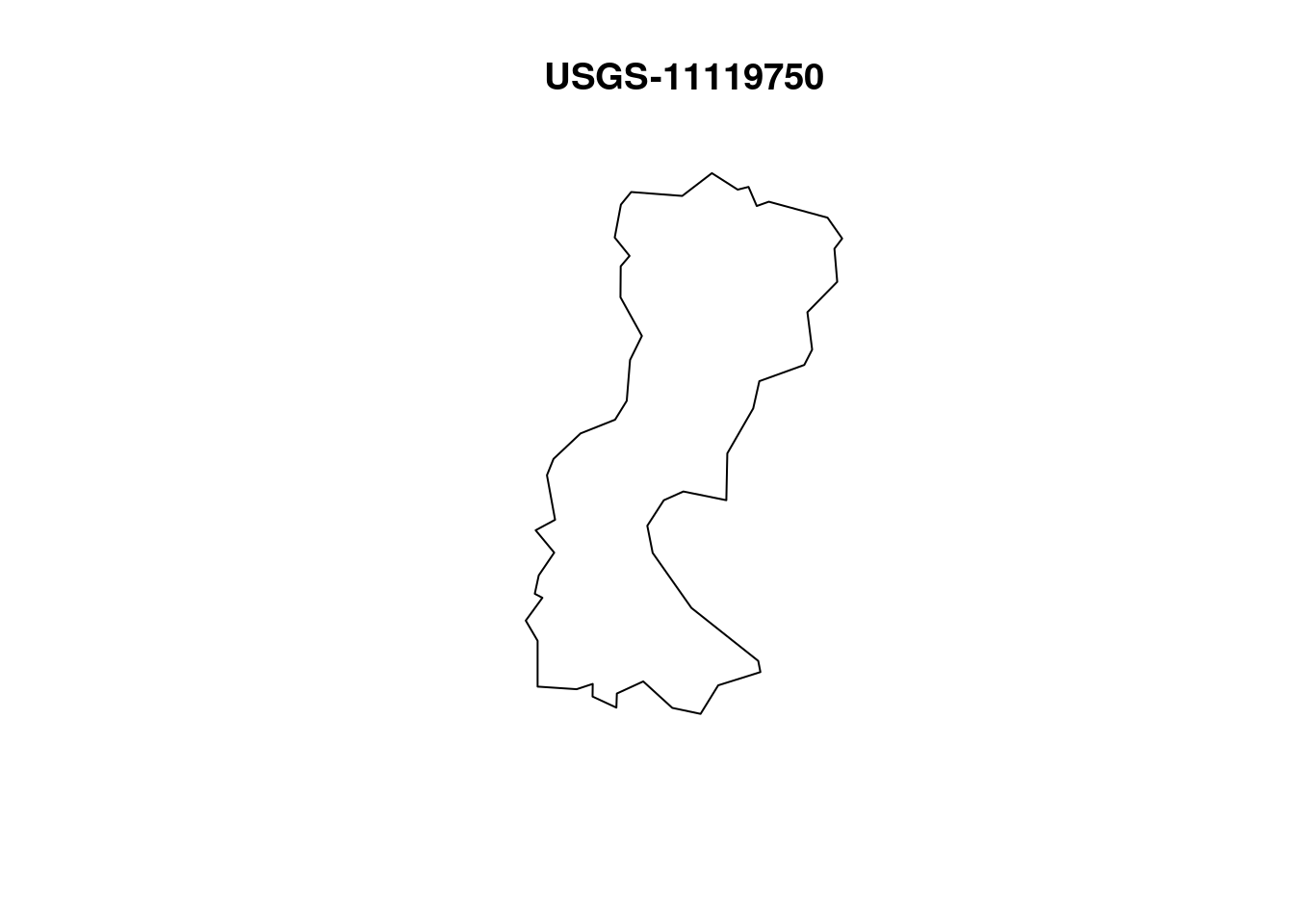# Collecting Data

We begin this lab by collecting data from two sources: the USGS, and OpenStreetMap. Here we see the AOI, and corresponding data that we will use.

``````# Getting Basin Boundary
basin_boundary_elev <- elevatr::get_elev_raster(basin_boundary, z = 13) %>%
raster::crop(basin_boundary) %>%

basin_boundary_elev <- basin_boundary_elev * 3.281 # convert `m` to `ft`

# Comment when file is already written
# raster::writeRaster(basin_boundary_elev, "data/basin-boundary-elev.tif", overwrite = TRUE)

bb <- st_bbox(basin_boundary) %>%
st_as_sfc() %>%
st_transform(4326)

# OSM Data
basin_buildings <- osmdata::opq(bb) %>%
osmdata_sf()

basin_buildings <- basin_buildings\$osm_polygons %>%
sf::st_centroid() %>%
st_intersection(basin_boundary)

# Plot to test if clipping worked as intended
# plot(basin_boundary)
# plot(basin_buildings, max.plot=1, add = TRUE)

basin_railway <- dplyr::filter(basin_buildings, amenity == "railway")

basin_streams <- osmdata::opq(bb) %>%
add_osm_feature(key = "waterway", value = "stream") %>%
osmdata_sf()

basin_streams <- basin_streams\$osm_lines %>%
st_intersection(basin_boundary)

# Plot to test if clipping worked as intended
# plot(basin_boundary)
# plot(basin_streams, max.plot=1, add = TRUE)``````

### Basin Boundary